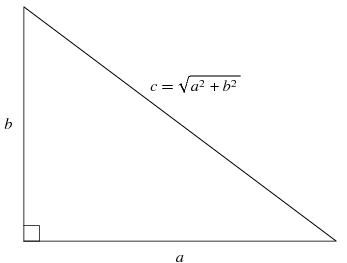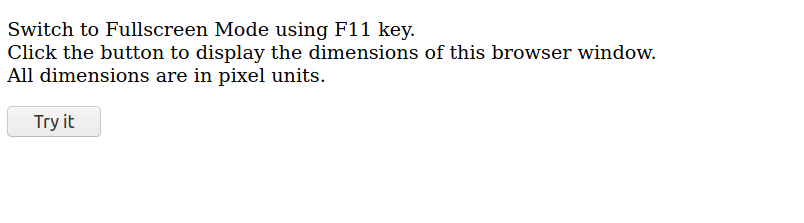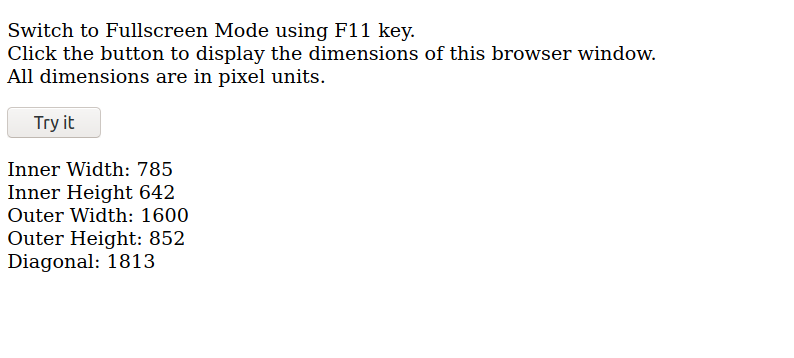# How to get the diagonal length of the device screen using JavaScript ?

Knowing the width and height of a browser window helps a web developer to enhancing the user experience. It can help in improving browser animations and the relative positioning of divisions and containers. Javascript provides a window object that represent an open browser window. It provides the various properties which define the dimensions of your browser window. They are as follows:

• innerWidth: It returns the width of the window’s content area.
• innerHeight: It returns the height of the window’s content area.
• outerWidth: It returns the width of the browser window.
• outerHeight: It returns the height of the browser window.

Note: These dimensions are in pixels.

Since pixels are a dimensionally square unit. You cannot tell accurately how many pixels lie on the diagonal. You can approximate the diagonal by using the browser height and width and applying the Pythagoras theorem.

Pythagoras Theorem: For a right-angled triangle, hypotenuse squared is the sum of base squared and height squared.Analogous to the above formula, Diagonal = Squared_root(Width^2 + Height^2).

Code Snippet:

 ` `

The above code will print the browser height and width in the console window. The console window can be opened using the developer tools in your browser.
Note: To get the complete screen dimensions, switch your browser to fullscreen mode. Most browsers switch the fullscreen mode using the F11 key.

The below code displays the results on the browser window using the innerHTML property of Javascript.

 ` ` `<``html``> ` ` `  `<``body``> ` ` `  `    ``<``p``> ` `        ``Switch to Fullscreen Mode using the F11 key. ` `        ``<``br``>Click the button to display the dimensions  ` `        ``of this browser window.<``br``> All dimensions  ` `        ``are in pixel units. ` `    `` ` ` `  `    ``<``button` `onclick``=``"myFunction()"``>Try it ` ` `  `    ``<``p` `id``=``"demo"``> ` ` `  `    ``<``script``> ` `        ``function myFunction() { ` `            ``var i_w = window.innerWidth; ` `            ``var i_h = window.innerHeight; ` `            ``var o_w = window.outerWidth; ` `            ``var o_h = window.outerHeight; ` `            ``var d = Math.sqrt(o_w * o_w + o_h * o_h); ` ` `  `            ``document.getElementById("demo").innerHTML ` `                ``= "Inner Width: " + i_w + ` `                ``"<``br``>Inner Height " + i_h + "<``br``>Outer Width: " ` `                ``+ o_w + "<``br``>Outer Height: " + ` `                ``o_h + "<``br``>Diagonal: " + Math.ceil(d); ` `        ``} ` `    `` ` ` ` ` `  ` `

Output:

• Before pressing ‘Try It’ button:• After pressing ‘Try It’ button:Note: If you know the linear pixel density of your monitor screen, you can divide the dimensions obtained from the above code with the density to get the dimensions in centimeters or inches.My Personal Notes arrow_drop_upCheck out this Author's contributed articles.

If you like GeeksforGeeks and would like to contribute, you can also write an article using contribute.geeksforgeeks.org or mail your article to contribute@geeksforgeeks.org. See your article appearing on the GeeksforGeeks main page and help other Geeks.

Please Improve this article if you find anything incorrect by clicking on the "Improve Article" button below.